# Math in Focus Grade 3 Chapter 10 Practice 5 Answer Key Subtraction

Practice the problems of Math in Focus Grade 3 Workbook Answer Key Chapter 10 Practice 5 Subtraction to score better marks in the exam.

## Math in Focus Grade 3 Chapter 10 Practice 5 Answer Key Subtraction

Complete each number bond. Then subtract.

Example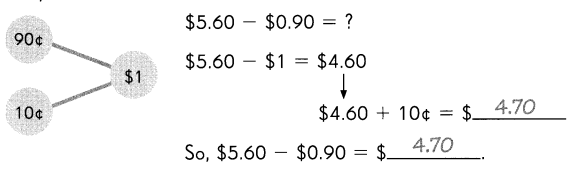Question 1.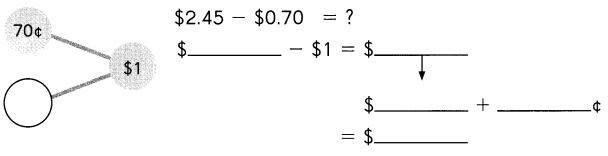So, $2.45 –$0.70 = $______________ Answer: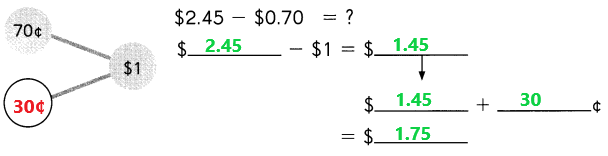So,$2.45 – $0.70 =$1.75
Explanation:
To make $0.70 to the nearest integer we have to add required ¢ to the$0.70.
A number bond is a simple addition of two numbers that add up to give the sum. Add these two numbers 70¢ and 30¢ the sum is $1. In place of$0.70 we have to use $1. Subtract$1 from $2.45 the difference is$1.45.
The 30¢ which are added to the given number $0.70 to make nearest integer are added to the above difference. Add$1.45 with 30¢ the sum is $1.75. Question 2.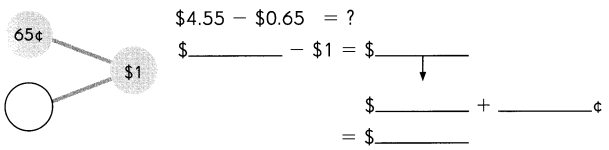So,$4.55 – $0.65 =$ ______________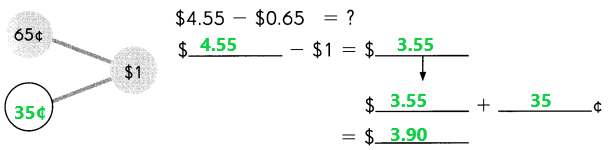So, $4.55 –$0.65 = $3.90 Explanation: To make$0.65 to the nearest integer we have to add required ¢ to the $0.65. A number bond is a simple addition of two numbers that add up to give the sum. Add these two numbers 65¢ and 35¢ the sum is$1.
In place of $0.65 we have to use$1. Subtract $1 from$4.55 the difference is $3.55. The 35¢ which are added to the given number$0.65 to make nearest integer are added to the above difference.
Add $3.55 with 35¢ the sum is$3.90.

Question 3.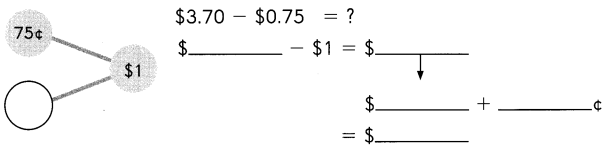So, $3.70 –$0.75 = $______________ Answer: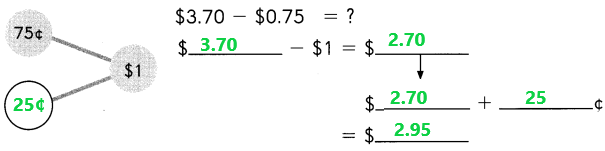So,$3.70 – $0.75 =$2.95
Explanation:
To make $0.75 to the nearest integer we have to add required ¢ to the$0.75.
A number bond is a simple addition of two numbers that add up to give the sum. Add these two numbers 75¢ and 25¢ the sum is $1. In place of$0.75 we have to use $1. Subtract$1 from $3.70 the difference is$2.70.
The 25¢ which are added to the given number $0.75 to make nearest integer are added to the above difference. Add$2.70 with 25¢ the sum is $2.95. Complete each number bond. Then subtract. Example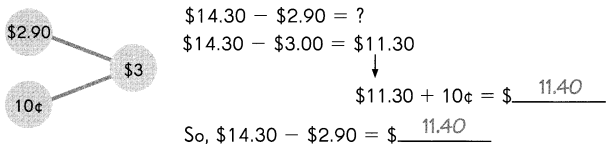Question 4.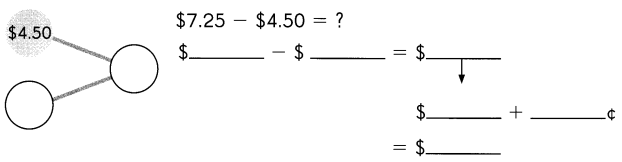So,$7.25 – $4.50 =$___________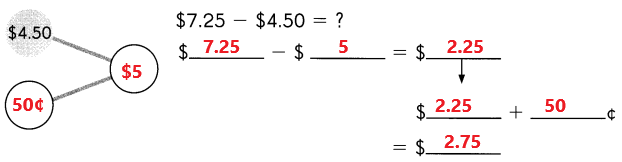So, $7.25 –$4.50 = $2.75 Explanation: To make$4.50 to the nearest integer we have to add required ¢ to the $4.50. A number bond is a simple addition of two numbers that add up to give the sum. Add these two numbers$4.50 and 50¢ the sum is $5. In place of$4.50 we have to use $5. Subtract$5 from $7.25 the difference is$2.25.
The 50¢ which are added to the given number $4.50 to make nearest integer are added to the above difference. Add$2.25 with 50¢ the sum is $2.75. Question 5.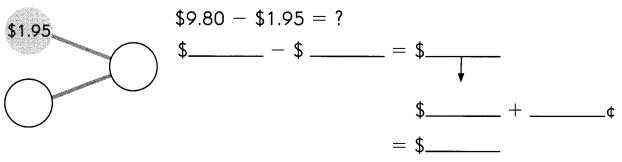So,$9.80 – $1.95 =$____________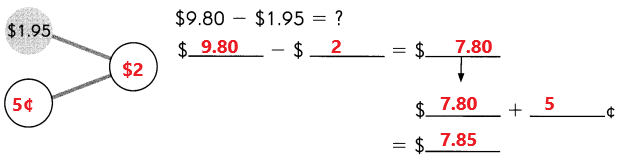So, $9.80 –$1.95 = $7.85 Explanation: To make$1.95 to the nearest integer we have to add required ¢ to the $1.95. A number bond is a simple addition of two numbers that add up to give the sum. Add these two numbers$1.95 and 5¢ the sum is $2. In place of$1.95 we have to use $2. Subtract$2 from $9.80 the difference is$7.80.
The 5¢ which are added to the given number $1.95 to make nearest integer are added to the above difference. Add$7.80 with 5¢ the sum is $7.85. Question 6.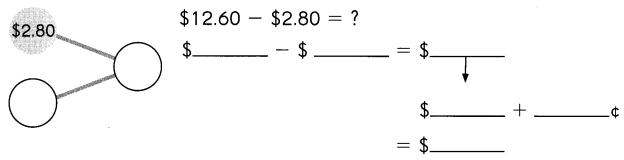So,$12.60 – $2.80 =$___________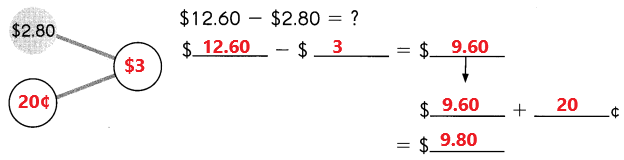So, $12.60 –$2.80 = $9.80 Explanation: To make$2.80 to the nearest integer we have to add required ¢ to the $2.80. A number bond is a simple addition of two numbers that add up to give the sum. Add these two numbers$2.80 and 20¢ the sum is $3. In place of$2.80 we have to use $3. Subtract$3 from $12.60 the difference is$9.60.
The 20¢ which are added to the given number $2.80 to make nearest integer are added to the above difference. Add$9.60 with 20¢ the sum is \$9.80.